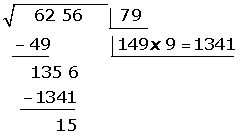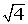## Exercise 1.

Look for the term unknown and indicate its name in the following operations:

1.
327 + ....... = 1,208

2.
....... – 4,121 = 626

3.
321 · ....... = 32,100

4.
28,035 : ....... = 623

## Exercise 2.

Look for the term unknown in the following operations:

1.
4 · (5 + ...) = 36

2.
(30 – ...) : 5 + 4 = 8

3.
18 · ... + 4 · ... = 56

4.
30 - ... : 8 = 25

## Exercise 3.

Calculate two different ways of completing the following operations:

1.
17 · 38 + 17 · 12 =

2.
6 · 59 + 4 · 59 =

3. (6 + 12) : 3

## Exercise 4.

Remove the common factor:

1.
7 · 5 - 3 · 5 + 16 · 5 - 5 · 4 =

2.
6 · 4 - 4 · 3 + 4 · 9 - 5 · 4 =

3. 8 · 34 + 8 · 46 + 8 · 20 =

## Exercise 5.

Express the follwing numbers in powers:

1.
50,000

2.
3,200

3.
3,000,000

Calculate:

1.
3³ · 34 · 3 =

2.
57 : 5³ =

3.
(5³)4 =

4.
(5 · 2 · 3)4 =

5.
(34)4 =

6.
[(5³)4 ]² =

7.
(8²)³

8.
(9³)²

9.
25 · 24 · 2 =

10. 27 : 26 =

11.
(2²)4 =

12.
(4 · 2 · 3)4 =

13.(25)4 =

14.
[(2³)4]0=

15.
(27²)5=

16.
(4³)² =

## Exercise 7.

Using powers, carry out the polynomial decomposition of these numbers:

1.
3,257

2.
10,256

3. 125,368

## Exercise 8.

Calculate the square roots:

1.2.3.## Exercise 9.

Calculate:

1.
27 + 3 · 5 – 16 =

2.
27 + 3 – 45 : 5 + 16 =

3.
(2 · 4 + 12) (6 − 4) =

4.
3 · 9 + (6 + 5 – 3) – 12 : 4 =

5.
2 + 5 · (2 · 3)³ =

6.
440 − [30 + 6 (19 − 12)] =

7.

2{4 [7 + 4 (5 · 3 − 9)] − 3 (40 − 8)} =

8.
7 · 3 + [6 + 2 · (2³ : 4 + 3 · 2) – 7 ·] + 9 : 3 =

## Solution of exercise 1

Look for the unknown term and indicate its name in the following operations:

1.
327 + ....... = 1,208

1,208 − 327 = 881

2.
....... – 4,121 = 626

Minuend.

4,121 + 626 = 4,747

3.
321 · ....... = 32 100

Factor.

32,100 : 321 = 100

4.
28,035: ....... = 623

Divisor .

28,035 : 623 = 45

## Solution of exercise 2

Look for the unknown term in the following operations:

1.
4 · (5 + ...) = 36

4

2.
(30 – ...) : 5 + 4 = 8

10

3.
18 · ... + 4 · ... = 56

2 and 5

4.
30 - ... : 8 = 25

40

## Solution of exercise 3

Calculate two different ways of completing the following operations:

1.
17 · 38 + 17 · 12 =

1.
17 · 38 + 17 · 12 = 646 + 204 = 850

2.
17 · 38 + 17 · 12 = 17 (38 + 12) = 17 · 50 = 850

2.
6 · 59 + 4 · 59 =

1.
6 · 59 + 4 · 59 = 354 + 236 = 590

2.
6 · 59 + 4 · 59 = 59 (6 + 4) = 59 · 10 = 590

3. (6 + 12) : 3

1.(6 + 12) : 3 = 18 : 3 = 6

2.(6 + 12) : 3 = (6 : 3) + (12 : 3) = 2 + 4 = 6

## Solution of exercise 4

Remove the common factor:

1.
7 · 5 - 3 · 5 + 16 · 5 - 5 · 4 =

7 · 5 - 3 · 5 + 16 · 5 - 5 · 4 = 5 (7 − 3 + 16 − 4)

2.
6 · 4 - 4 · 3 + 4 · 9 - 5 · 4 =

6 · 4 - 4 · 3 + 4 · 9 - 5 · 4 = 4 (6 − 3 + 9 − 5)

3. 8 · 34 + 8 · 46 + 8 · 20 =

8 · (34 + 46 + 20)

## Solution of exercise 5

Express the following numbers in powers:

1.
50,000 = 5 · 10 4

2.
3,200 =
32 · 10 2

3.
3,000,000 =
3 · 10 6

## Solution of exercise 6

Calculate:

1.
3³ · 34 · 3 = 38

2.
57 : 5³ = 54

3.
(5³)4 = 512

4.
(5 · 2 · 3) 4 = 304

5.(34)4 = 316

6.
[(5³)4]² = (512)² = 524

7.
(8²)³ =[( 2³)²]³ = (26)³ = 218

8.
(9³)² = [(3²)³]² = (36)² = 312

9.
25 · 24 · 2 = 210

10. 27 : 26 = 2

11.
(2²)4 = 28

12.
(4 · 2 · 3)4 = 244

13.(25)4 = 220

14.
[(2³)4]0 = (212)0 = 20 = 1

15.
(27²)5 =[(3³)²]5 = (36)5 = 330

16.
(4³)² = [(2²)³]² = (26)² = 212

## Solution of exercise 7

Using powers, carry out the polynomial decomposition of these numbers:

1.
3,257

3,257 = 3 · 10³ + 2 · 10² + 5 · 10 + 7

2.
10,256

10,256 = 1 · 104 + 0 · 10³ + 2 · 10² + 5 · 10 + 6

3.
125,368

125,368 = 1 · 105 + 2 · 104 +5 · 10³ + 3 · 10² + 6 · 10 + 8

## Solution of exercise 8

Calculate the square roots:

1.2.3.## Solution of exercise 9

Calculate:

1.
27 + 3 · 5 – 16 =

= 27 + 15 − 16 = 26

2.
27 + 3 – 45 : 5 + 16=

27 + 3 – 9 + 16 = 37

3.
(2 · 4 + 12) (6 − 4) =

= (8 + 12) (2) = 20 · 2 = 40

4.
3 · 9 + (6 + 5 – 3) – 12 : 4 =

= 27 + 8 – 3 = 32

5.
2 + 5 · (2 ·3)³ =

= 2 + 5 · (6)³ = 2 + 5 · 216 = 2 + 1080 = 1082

6.
440 − [30 + 6 (19 − 12)] =

= 440 − (30 + 6 · 7)] = 440 − (30 + 42) =

= 440 − (72) = 368

7.
2{4[7 + 4 (5 · 3 − 9)] − 3 (40 − 8)} =

= 2[4 (7 + 4 · 6) − 3 (32)] = 2[4 (7 + 24) − 3 (32)]=

2[4 (31) − 3 (32)]= 2 (124 − 96)= 2 (28)= 56

8.7 · 3 + [6 + 2 · (2³ : 4 + 3 · 2) – 7 ·] + 9 : 3 =

= 21 + [ 6 + 2 · (2+ 6) – 14] +3 =

= 21 + ( 6 + 2 · 8 – 14) +3 =

= 21 + ( 6 + 16 – 14) + 3 =

= 21 + 8 + 3 = 32

Did you like the article?(No Ratings Yet)Loading...

Emma

I am passionate about travelling and currently live and work in Paris. I like to spend my time reading, gardening, running, learning languages and exploring new places.

Did you like
this resource?

Bravo!